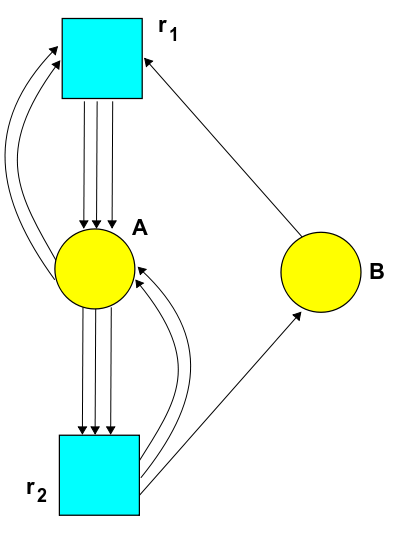# The Azimuth Project Experiments in multiple equilibrium states (Rev #1, changes)

Showing changes from revision #0 to #1: Added | Removed | Changed

## Example from Feinberg

We consider examples from Table 1 in the following paper.

P. M. Schlosser and M. Feinberg, A theory of multiple steady states in isothermal homogeneous CFSTRs with many reactions, Chemical Engineering Science 49 (1994), 1749–1767.

The example we consider here is from Table 1 (7).

$2 A + B \to 3 A$
$3 A \to 2 A + B$

By ‘just one equilibrium state’, I presume they mean just a 1-parameter family of equilibrium states. After all, if $x_A, x_B$ is an equilibrium solution of the rate equation, so is $c x_A, c x_B$ for any $c \ge 0$.

### Example of multiple equilibrium states

Consider the chemical reaction network defined by

$2 A + B \to 3 A$
$3 A \to 2 A + B$

The input and output functions then give.

$m(\tau_1)=\{2,1\}$
$n(\tau_1)=\{3,0\}$

and for $\tau_2$

$m(\tau_2)=\{3,0\}$
$n(\tau_2)=\{2,1\}$

### The Stochastic Petri Net### The chemical rate equation

The general form of the rate equation is

$\frac{d}{d t}X_i=\sum_{\tau\in T}r(\tau)X^{m(\tau)}(n_i(\tau)-m_i(\tau))$

The population of species $A$ ($B$) will be denoted $X_1(t)$ ($X_2(t)$) and the rate of the reaction $\tau_1$ ($\tau_2$) as $r_1$ ($r_2$).

$\frac{d}{d t}X_1 = r_1 X_1^2 X_2 - r_2 X_1^3$

and

$\frac{d}{d t} X_2 = r_1 X_1^2 X_2 + r_2 X_1^3$

### The master equation

The general form of the master equation is

$H = \sum_{\tau\in T} r(\tau)(a^\dagger^{n(\tau)}a^{m(\tau)} - a^\dagger^{m(\tau)} a^{m(\tau)})$

In our case, this becomes

$H = r_1 (a_1^\dagger a_1^\dagger a_1^\dagger a_1 a_1 a_2 - a_1^\dagger a_1^\dagger a_2^\dagger a_1 a_1 a_2) + r_2 (a_1^\dagger a_1^\dagger a_2^\dagger a_1 a_1 a_1 - a_1^\dagger a_1^\dagger a_1^\dagger a_1 a_1 a_1 )$

We consider a wave function of the form

$\Psi := e^{b z_1} e^{c z_2}$

We then calculate $H\Psi$. We find solutions where this vanishes, for non-zero $\Psi$ as

$(r_1 b^2 c - r_2 b^3)z_1^3 + (r_2 b^3 - r_1 b^2 c)z_1^2 z_2$

For this to vanish, each of the terms must vanish separately. Hence we arrive at a system of equations

$r_1 b^2 c - r_2 b^3 = 0$
$r_2 b^3 - r_1 b^2 c = 0$

In this case, and in every case I’ve considered so far, a solution of one of these equations, gives a solution of the second.